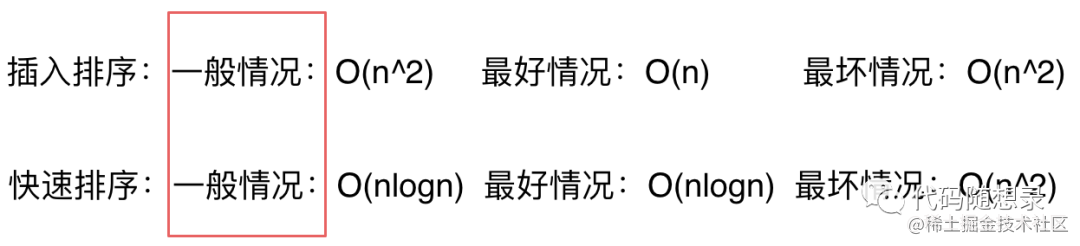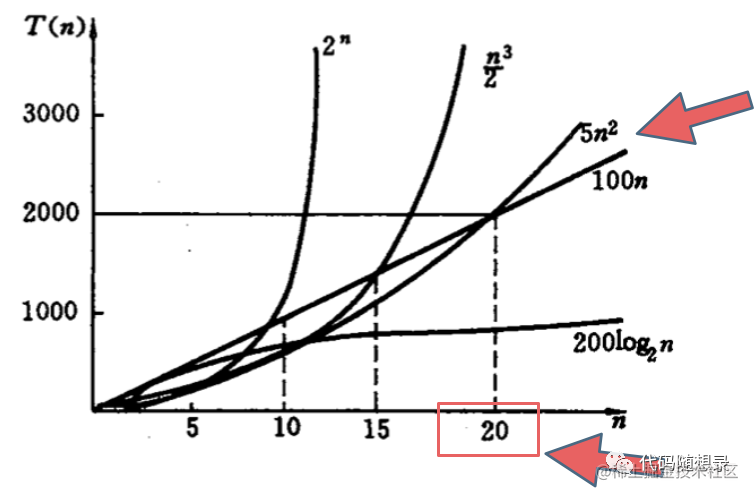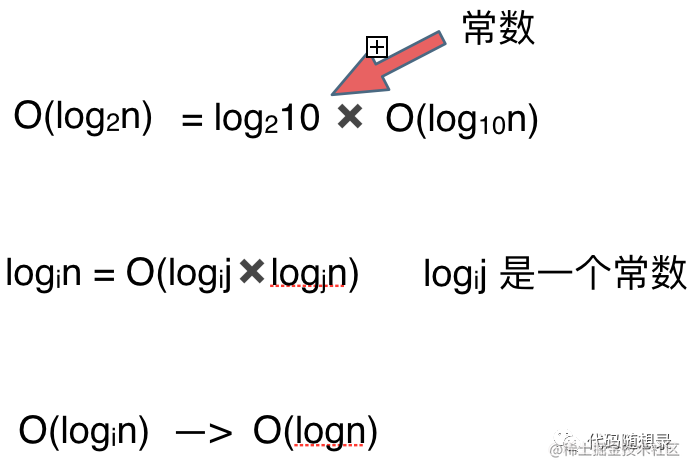# 究竟什么是时间复杂度，怎么求时间复杂度，看这一篇就够了

（给 算法爱好者加星标，修炼编程内功 ）

# 什么是大O# 如何描述时间复杂度`O(1)常数阶 < O(logn)对数阶 < O(n)线性阶 < O(n^2)平方阶 < O(n^3)(立方阶) < O(2^n) (指数阶)`

# 你所不知道的O(logn)# 如果时间复杂度是一个复杂的表达式，我们如何简化

`O(2*n^2 + 10*n + 1000)`

`O(2*n^2 + 10*n)`

`O(n^2 + n)`

`O(n^2)`

`O(n^2)`

`O(3*n^2)`

`O(2*n^2 + 10*n + 1000) < O(3*n^2)`

# 举例说明时间复杂度要怎么算